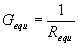Norton & Thevenin equivalent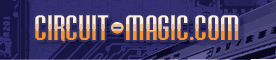Circuit analysis Tutorial
AKNM Circuit Magic- circuit analysis software

# Norton & Thevenin equivalent

Thévenin's Theorem

Any voltage network which may be viewed from two terminals can be replaced by a voltage-source equivalent circuit comprising a single voltage source E and a single series resistance R.. The voltage V is the open-circuit voltage between the two terminals and the resistance Z is the resistance of the network viewed from the terminals with all voltage sources removed from circuit.

Sample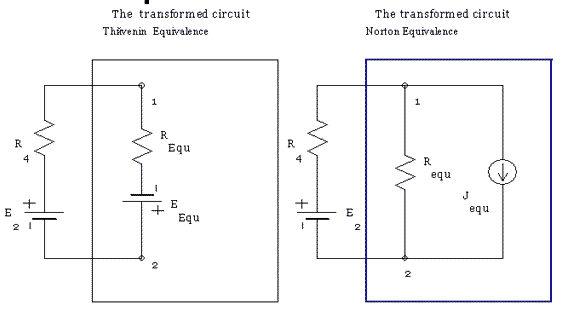All circuits are equivalent. Resistors R1,R2, R3 and voltage source are transformed into Requ Eequ,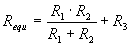see parallel, series simplifications.

To determine Eequ we shall break off branch connecting node 1 and node 2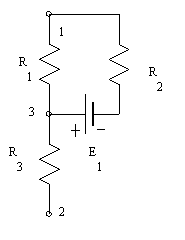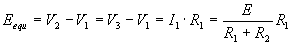Norton's Theorem

Any current network which may be viewed from two terminals can be replaced by a current-source equivalent circuit comprising a single current source I and a single shunt conductance G. The current I is the short-circuit current between the two terminals and the conductance G is the conductance of the network viewed from the terminals with all branches containing current sources are broken off.